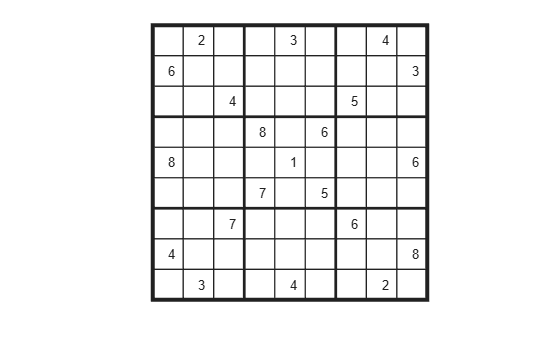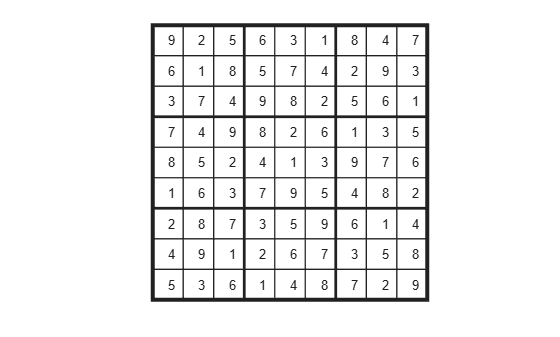Documentation

## Solve Sudoku Puzzles Via Integer Programming: Solver-Based

This example shows how to solve a Sudoku puzzle using binary integer programming. For the problem-based approach, see Solve Sudoku Puzzles Via Integer Programming: Problem-Based.

You probably have seen Sudoku puzzles. A puzzle is to fill a 9-by-9 grid with integers from 1 through 9 so that each integer appears only once in each row, column, and major 3-by-3 square. The grid is partially populated with clues, and your task is to fill in the rest of the grid.

### Initial Puzzle

Here is a data matrix `B` of clues. The first row, `B(1,2,2)`, means row 1, column 2 has a clue 2. The second row, `B(1,5,3)`, means row 1, column 5 has a clue 3. Here is the entire matrix `B`.

```B = [1,2,2; 1,5,3; 1,8,4; 2,1,6; 2,9,3; 3,3,4; 3,7,5; 4,4,8; 4,6,6; 5,1,8; 5,5,1; 5,9,6; 6,4,7; 6,6,5; 7,3,7; 7,7,6; 8,1,4; 8,9,8; 9,2,3; 9,5,4; 9,8,2]; drawSudoku(B) % For the listing of this program, see the end of this example.```This puzzle, and an alternative MATLAB® solution technique, was featured in Cleve's Corner in 2009.

There are many approaches to solving Sudoku puzzles manually, as well as many programmatic approaches. This example shows a straightforward approach using binary integer programming.

This approach is particularly simple because you do not give a solution algorithm. Just express the rules of Sudoku, express the clues as constraints on the solution, and then `intlinprog` produces the solution.

### Binary Integer Programming Approach

The key idea is to transform a puzzle from a square 9-by-9 grid to a cubic 9-by-9-by-9 array of binary values (0 or 1). Think of the cubic array as being 9 square grids stacked on top of each other. The top grid, a square layer of the array, has a 1 wherever the solution or clue has a 1. The second layer has a 1 wherever the solution or clue has a 2. The ninth layer has a 1 wherever the solution or clue has a 9.

This formulation is precisely suited for binary integer programming.

The objective function is not needed here, and might as well be 0. The problem is really just to find a feasible solution, meaning one that satisfies all the constraints. However, for tie breaking in the internals of the integer programming solver, giving increased solution speed, use a nonconstant objective function.

### Express the Rules for Sudoku as Constraints

Suppose a solution $x$ is represented in a 9-by-9-by-9 binary array. What properties does $x$ have? First, each square in the 2-D grid (i,j) has exactly one value, so there is exactly one nonzero element among the 3-D array entries $x\left(i,j,1\right),...,x\left(i,j,9\right)$. In other words, for every $i$ and $j$,

`$\sum _{k=1}^{9}x\left(i,j,k\right)=1.$`

Similarly, in each row $i$ of the 2-D grid, there is exactly one value out of each of the digits from 1 to 9. In other words, for each $i$ and $k$,

`$\sum _{j=1}^{9}x\left(i,j,k\right)=1.$`

And each column $j$ in the 2-D grid has the same property: for each $j$ and $k$,

`$\sum _{i=1}^{9}x\left(i,j,k\right)=1.$`

The major 3-by-3 grids have a similar constraint. For the grid elements $1\le i\le 3$ and $1\le j\le 3$, and for each $1\le k\le 9$,

`$\sum _{i=1}^{3}\sum _{j=1}^{3}x\left(i,j,k\right)=1.$`

To represent all nine major grids, just add 3 or 6 to each $i$ and $j$ index:

$\sum _{i=1}^{3}\sum _{j=1}^{3}x\left(i+U,j+V,k\right)=1,$ where $U,V\phantom{\rule{0.5em}{0ex}}ϵ\phantom{\rule{0.5em}{0ex}}\left\{0,3,6\right\}.$

### Express Clues

Each initial value (clue) can be expressed as a constraint. Suppose that the $\left(i,j\right)$ clue is $m$ for some $1\le m\le 9$. Then $x\left(i,j,m\right)=1$. The constraint $\sum _{k=1}^{9}x\left(i,j,k\right)=1$ ensures that all other $x\left(i,j,k\right)=0$ for $k\ne m$.

### Write the Rules for Sudoku

Although the Sudoku rules are conveniently expressed in terms of a 9-by-9-by-9 solution array `x`, linear constraints are given in terms of a vector solution matrix `x(:)`. Therefore, when you write a Sudoku program, you have to use constraint matrices derived from 9-by-9-by-9 initial arrays.

Here is one approach to set up Sudoku rules, and also include the clues as constraints. The `sudokuEngine` file comes with your software.

`type sudokuEngine`
```function [S,eflag] = sudokuEngine(B) % This function sets up the rules for Sudoku. It reads in the puzzle % expressed in matrix B, calls intlinprog to solve the puzzle, and returns % the solution in matrix S. % % The matrix B should have 3 columns and at least 17 rows (because a Sudoku % puzzle needs at least 17 entries to be uniquely solvable). The first two % elements in each row are the i,j coordinates of a clue, and the third % element is the value of the clue, an integer from 1 to 9. If B is a % 9-by-9 matrix, the function first converts it to 3-column form. % Copyright 2014 The MathWorks, Inc. if isequal(size(B),[9,9]) % 9-by-9 clues % Convert to 81-by-3 [SM,SN] = meshgrid(1:9); % make i,j entries B = [SN(:),SM(:),B(:)]; % i,j,k rows % Now delete zero rows [rrem,~] = find(B(:,3) == 0); B(rrem,:) = []; end if size(B,2) ~= 3 || length(size(B)) > 2 error('The input matrix must be N-by-3 or 9-by-9') end if sum([any(B ~= round(B)),any(B < 1),any(B > 9)]) % enforces entries 1-9 error('Entries must be integers from 1 to 9') end %% The rules of Sudoku: N = 9^3; % number of independent variables in x, a 9-by-9-by-9 array M = 4*9^2; % number of constraints, see the construction of Aeq Aeq = zeros(M,N); % allocate equality constraint matrix Aeq*x = beq beq = ones(M,1); % allocate constant vector beq f = (1:N)'; % the objective can be anything, but having nonconstant f can speed the solver lb = zeros(9,9,9); % an initial zero array ub = lb+1; % upper bound array to give binary variables counter = 1; for j = 1:9 % one in each row for k = 1:9 Astuff = lb; % clear Astuff Astuff(1:end,j,k) = 1; % one row in Aeq*x = beq Aeq(counter,:) = Astuff(:)'; % put Astuff in a row of Aeq counter = counter + 1; end end for i = 1:9 % one in each column for k = 1:9 Astuff = lb; Astuff(i,1:end,k) = 1; Aeq(counter,:) = Astuff(:)'; counter = counter + 1; end end for U = 0:3:6 % one in each square for V = 0:3:6 for k = 1:9 Astuff = lb; Astuff(U+(1:3),V+(1:3),k) = 1; Aeq(counter,:) = Astuff(:)'; counter = counter + 1; end end end for i = 1:9 % one in each depth for j = 1:9 Astuff = lb; Astuff(i,j,1:end) = 1; Aeq(counter,:) = Astuff(:)'; counter = counter + 1; end end %% Put the particular puzzle in the constraints % Include the initial clues in the |lb| array by setting corresponding % entries to 1. This forces the solution to have |x(i,j,k) = 1|. for i = 1:size(B,1) lb(B(i,1),B(i,2),B(i,3)) = 1; end %% Solve the Puzzle % The Sudoku problem is complete: the rules are represented in the |Aeq| % and |beq| matrices, and the clues are ones in the |lb| array. Solve the % problem by calling |intlinprog|. Ensure that the integer program has all % binary variables by setting the intcon argument to |1:N|, with lower and % upper bounds of 0 and 1. intcon = 1:N; [x,~,eflag] = intlinprog(f,intcon,[],[],Aeq,beq,lb,ub); %% Convert the Solution to a Usable Form % To go from the solution x to a Sudoku grid, simply add up the numbers at % each \$(i,j)\$ entry, multiplied by the depth at which the numbers appear: if eflag > 0 % good solution x = reshape(x,9,9,9); % change back to a 9-by-9-by-9 array x = round(x); % clean up non-integer solutions y = ones(size(x)); for k = 2:9 y(:,:,k) = k; % multiplier for each depth k end S = x.*y; % multiply each entry by its depth S = sum(S,3); % S is 9-by-9 and holds the solved puzzle else S = []; end ```

### Call the Sudoku Solver

`S = sudokuEngine(B); % Solves the puzzle pictured at the start`
```LP: Optimal objective value is 29565.000000. Cut Generation: Applied 1 strong CG cut, and 3 zero-half cuts. Lower bound is 29565.000000. Relative gap is 0.00%. Optimal solution found. Intlinprog stopped at the root node because the objective value is within a gap tolerance of the optimal value, options.AbsoluteGapTolerance = 0 (the default value). The intcon variables are integer within tolerance, options.IntegerTolerance = 1e-05 (the default value). ```
`drawSudoku(S)`You can easily check that the solution is correct.

### Function to Draw the Sudoku Puzzle

`type drawSudoku`
```function drawSudoku(B) % Function for drawing the Sudoku board % Copyright 2014 The MathWorks, Inc. figure;hold on;axis off;axis equal % prepare to draw rectangle('Position',[0 0 9 9],'LineWidth',3,'Clipping','off') % outside border rectangle('Position',[3,0,3,9],'LineWidth',2) % heavy vertical lines rectangle('Position',[0,3,9,3],'LineWidth',2) % heavy horizontal lines rectangle('Position',[0,1,9,1],'LineWidth',1) % minor horizontal lines rectangle('Position',[0,4,9,1],'LineWidth',1) rectangle('Position',[0,7,9,1],'LineWidth',1) rectangle('Position',[1,0,1,9],'LineWidth',1) % minor vertical lines rectangle('Position',[4,0,1,9],'LineWidth',1) rectangle('Position',[7,0,1,9],'LineWidth',1) % Fill in the clues % % The rows of B are of the form (i,j,k) where i is the row counting from % the top, j is the column, and k is the clue. To place the entries in the % boxes, j is the horizontal distance, 10-i is the vertical distance, and % we subtract 0.5 to center the clue in the box. % % If B is a 9-by-9 matrix, convert it to 3 columns first if size(B,2) == 9 % 9 columns [SM,SN] = meshgrid(1:9); % make i,j entries B = [SN(:),SM(:),B(:)]; % i,j,k rows end for ii = 1:size(B,1) text(B(ii,2)-0.5,9.5-B(ii,1),num2str(B(ii,3))) end hold off end ```